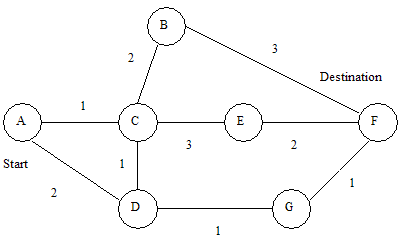## Posts Tagged ‘dijkstras’

### Dijkstra’s Algorithm code in C++

Tuesday, July 15th, 2008

Dijkstra’s algorithm is a famous algorithm that calculates the routes and distances from a start node to all other nodes in a connected graph where all the distances are positive.

Here is a version which I wrote in C++. My aim here was to try to create a version that was easy to read, rather than the most efficient version. I used the excellent Introduction to Algorithms book as a reference for my version of Dijkstra’s. This version is implemented using STL vectors to store the edges and nodes. I might later modify it to use adjacency lists and priority queues to produce a more efficient implementation.

First off is my `Node` and `Edge` class. You’ll note that the objects add themselves to the `nodes` and `edges` vectors as they are constructed.

```vector<Node*> nodes;
vector<Edge*> edges;
class Node
{
public:
Node(char id)
: id(id), previous(NULL),
distanceFromStart(INT_MAX)
{
nodes.push_back(this);
}
public:
char id;
Node* previous;
int distanceFromStart;
};
class Edge
{
public:
Edge(Node* node1, Node* node2, int distance)
: node1(node1), node2(node2), distance(distance)
{
edges.push_back(this);
}
bool Connects(Node* node1, Node* node2)
{
return (
(node1 == this->node1 &&
node2 == this->node2) ||
(node1 == this->node2 &&
node2 == this->node1));
}
public:
Node* node1;
Node* node2;
int distance;
};
```

Next up is my code to construct a simple test graph. The starting node is initialised with a distance of 0. Dijkstra’s algorithm is then called before the results are printed out. Underneath the code is a diagram of what this graph looks like.

```void DijkstrasTest()
{
Node* a = new Node('a');
Node* b = new Node('b');
Node* c = new Node('c');
Node* d = new Node('d');
Node* e = new Node('e');
Node* f = new Node('f');
Node* g = new Node('g');
Edge* e1 = new Edge(a, c, 1);
Edge* e2 = new Edge(a, d, 2);
Edge* e3 = new Edge(b, c, 2);
Edge* e4 = new Edge(c, d, 1);
Edge* e5 = new Edge(b, f, 3);
Edge* e6 = new Edge(c, e, 3);
Edge* e7 = new Edge(e, f, 2);
Edge* e8 = new Edge(d, g, 1);
Edge* e9 = new Edge(g, f, 1);
a->distanceFromStart = 0; // set start node
Dijkstras();
PrintShortestRouteTo(f);
// Node / Edge memory cleanup snipped
}
```Here is the actual algorithm implementation. We remove the node with the smallest distance from the list of nodes, and then calculate all the minimum distances. We repeat until there are no more nodes left.

```void Dijkstras()
{
while (nodes.size() > 0)
{
Node* smallest = ExtractSmallest(nodes);
for (int i=0; i<size; ++i)
{
int distance = Distance(smallest, adjacent) +
smallest->distanceFromStart;
{
}
}
}
}
```

Here are the supporting functions. The first function removes and returns the node with the smallest distance from the list.

The next returns a new vector containing all the nodes which are adjacent to the node that we pass in.

The third returns the distance value of an edge which directly connects the two nodes.

The final one checks if a node is in the list of nodes.

```// Find the node with the smallest distance,
// remove it, and return it.
Node* ExtractSmallest(vector<Node*>& nodes)
{
int size = nodes.size();
if (size == 0) return NULL;
int smallestPosition = 0;
Node* smallest = nodes.at(0);
for (int i=1; i<size; ++i)
{
Node* current = nodes.at(i);
if (current->distanceFromStart <
smallest->distanceFromStart)
{
smallest = current;
smallestPosition = i;
}
}
nodes.erase(nodes.begin() + smallestPosition);
return smallest;
}
// Return all nodes adjacent to 'node' which are still
// in the 'nodes' collection.
{
const int size = edges.size();
for(int i=0; i<size; ++i)
{
Edge* edge = edges.at(i);
if (edge->node1 == node)
{
}
else if (edge->node2 == node)
{
}
{
}
}
}
// Return distance between two connected nodes
int Distance(Node* node1, Node* node2)
{
const int size = edges.size();
for(int i=0; i<size; ++i)
{
Edge* edge = edges.at(i);
if (edge->Connects(node1, node2))
{
return edge->distance;
}
}
return -1; // should never happen
}
// Does the 'nodes' vector contain 'node'
bool Contains(vector<Node*>& nodes, Node* node)
{
const int size = nodes.size();
for(int i=0; i<size; ++i)
{
if (node == nodes.at(i))
{
return true;
}
}
return false;
}
```

And finally here is the code which prints out the route between our start node ‘a’ and the destination node ‘f’. It starts at the destination node and then works backwards using the previous pointer in each node.

```void PrintShortestRouteTo(Node* destination)
{
Node* previous = destination;
cout << "Distance from start: "
<< destination->distanceFromStart << endl;
while (previous)
{
cout << previous->id << " ";
previous = previous->previous;
}
cout << endl;
}
```

Update: 7th September 2008

I have now uploaded a zip file containing the Visual C++ Express Solution file and source code for this project – Dijkstra’s Source Code (8kb).